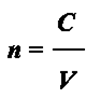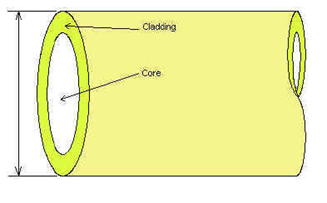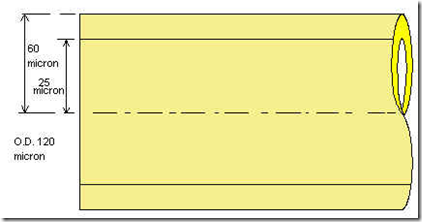# Fiber Optic Theory (1)

Reflection and Refraction of Light

When light travelling in a transparent material meets the surface of another transparent material two things happen

• 1. some of the light is reflected
• 2. some of the light is transmitted into the second transparent material

The light which is transmitted usually changes direction when it enters the second material. This bending of light is called refraction and it depends upon the fact that light travels at one speed in one material and at a different speed in a different material. As a result each material has its own Refractive Index which we use to help us calculate the amount of bending which takes place. Refractive index is defined as:where

n is the refractive index
C is the speed of light in a vacuum
V is the speed of light in the material

Two possible cases exist.

These are:

1. where light goes from a material with a low refractive index to one with a high refractive index, OR
2. where light goes from a material with a high refractive index to one with a low refractive index.

These two cases are shown in the diagrams below.

Figure 1 n1 < n2                                                               Figure 2 n1 > n2

Total Internal Reflection

In the second case above, θ 2 is always greater than θ 1 . So, as we increase θ1, eventually θ2 will reach 90o before θ 1 does. At this point where θ1has reached a value called the critical angle (θ c ).

The transmitted ray now tries to travel in both materials simultaneously For various reasons this is physically impossible so there is no transmitted ray and all the light energy is reflected. This is true for any value of θ1, the angle of incidence, equal to or greater than θ c This phenomenon is called Total Internal Reflection (TIR).

We can define the two conditions necessary for TIR to occur

1. The refractive index of the first medium is greater than the refractive index of the second one.

2. The angle of incidence, θ1, is greater than or equal to the critical angle, θc

The phenomenon of TIR causes 100% reflection. In no other situation in nature, where light is reflected, does 100% reflection occur. So TIR is unique and very useful.

Structure of Fiber

Optical fibers are very fine fibers of glass. They consist of a glass core, roughly fifty micrometres in diameter, surrounded by a glass "optical cladding" giving an outside diameter of about 120 micrometres. They make use of TIR to confine light within the core of the fiber.Figure 3 Structure of an Optical Fiber

The Structure of a Fiber

The core has a higher refractive index than the cladding. Although the cladding does not carry light, it is nevertheless an essential part of the fiber. The cladding is not just a mere covering. It keeps the value of the critical angle constant throughout the whole length of the fiber.Figure 4 Structure of a Fiber

Optical fibers are optical waveguides. This means that wherever the fiber goes the light, which is confined to the core of the fiber, also goes. So optical fibers can be used to make light bend round corners.